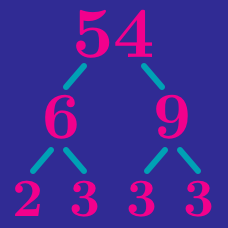Number Theory

Perfect Squares, Cubes and Powers

Which of the following is NOT a perfect square number?

If $270x$ is a perfect square, what is the smallest possible integer value of $x?$

Divide $297$ by the smallest possible positive integer $a$ so that the result is the square of a positive integer $b$. What is $a+b$?

Find the smallest positive integer $c$ that satisfies

$360a = 1500b = c^2,$

where $a$ and $b$ are positive integers.

Find the largest 4-digit positive integer which is a perfect power of 2.

×